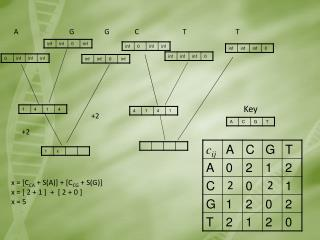DownloadDownload PresentationKey

# Key

Télécharger la présentation## Key

- - - - - - - - - - - - - - - - - - - - - - - - - - - E N D - - - - - - - - - - - - - - - - - - - - - - - - - - -
##### Presentation Transcript

1. A G G C T T Key +2 +2 x = [CCA + S(A)] + [CCG + S(G)] x = [ 2 + 1 ] + [ 2 + 0 ] x = 5 2 2

2. A G G C T T

3. Sankoff Summary • The algorithm is run on all the sites in the sequences • The result is the minimal cost for evolution of the data set on the tree S1 ACCCCTTC S2 ACCCCATA S3 ACTGCTTC S4 ACTGCTAA

4. Large Parsimony Problem • Finding the parsimony of several sequences considered an NP-Hard problem. • NP-Hard is used for credit card encryption • Calculations for 10 species requires more than 20 million trees. (20 species = 2.2E1020) • Fitch/Sankoff must be run on each tree

5. Tree Searching Methods • Exact Search • Precise, but for very small trees • Branch and Bound • Precise up to ~20 taxa • Heuristic Searches • Not precise, but allows larger inputs

6. Exact Search

7. Branch and Bound • Similar to Exact Search • Adds branches one at a time • Only follows paths with a parsimony score lower than the global score

8. Branch and Bound • Following the left sub tree gives us a global minimum of 9 • Middle Branch can be ignored • Following the right sub tree finds the optimal solution

9. Heuristic Searching • Is not guaranteed to find the optimal solution • Finds the optimal sub tree solution • Local peak phenomenon • Methods include: • Star Decomposition • Nearest Neighbor Interchanges • Subtree Pruning and Regrafting

10. Star Decomposition • The graph can be represented in star form • Each leg is lengthened • Each new graph is scored and the lowest parsimony is selected • The remaining edges are expanded

11. Nearest Neighbor Interchanges • Removes an internal edge and reconnects the split sub trees • Calculates the parsimony of the resulting graph

12. Tree Pruning and Regrafting Select a random edge Remove the edge from the graph Select another edge in the remaining graph Add the severed nodes back into the graph at that location Calculate the new parsimony scores

13. Summary • Tree Creation Methods • Evolutionary Scoring • Precise Searches • Heuristic Searches

14. Available Software • Mega4 • Elegantly handles downloading sequences, aligning them and then estimating and drawing phylogenetic trees • PAML • A package containing many tree construction and data analysis tools • PHYML • Useful for estimation of Maximum Likelihood trees • MrBayes • Used for Bayesian Inference of phyloenetic trees

15. Demonstration!